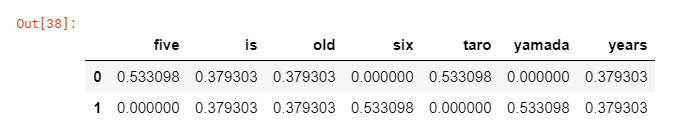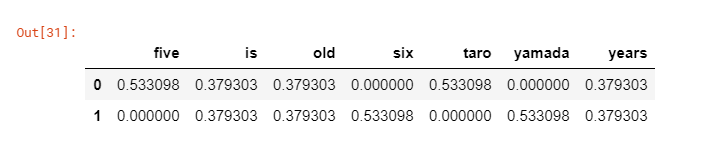Trigger

With the item getter that appeared in Mercari Competition 1st Code I didn't really understand TfidfVectorizer.

Summary

itemgetter → Arbitrary elements can be extracted from iterables (objects that can be written in in of for statements such as lists and character strings).

TfidfVectorizer → Used when calculating important characters in a sentence. The higher the score, the higher the frequency of appearance in the document, indicating that the characters are less likely to appear in other documents.

itemgetter

The callable object that gets the name element is returned in the first line. Only the name element is extracted in the second line.

qiita.rb

from operator import itemgetter

item=itemgetter("name")TfidfVectorizer

It is used to calculate TF-IDF. TF-IDF is used to identify important strings in a document. In the competition, it is used to quantify the brand names of the products on display. In the example below, five, six, taro, and yamada appear frequently and do not appear in other documents, so the score is high.

qiita.rb

import numpy as np
from sklearn.feature_extraction.text import TfidfVectorizer as Tfidf

sample_string= np.array(['taro is five years old','yamada is six years old'])
tfidf=Tfidf(max_features=100000, token_pattern='\w+')
x=tfidf.fit_transform(sample_string)

pd.DataFrame(y.toarray(), columns=tfidf.get_feature_names())Combined use of itemgetter, TfidfVectorizer, Pipeline

It pipelines an instance of itemgetter ("name") and an instance of Tfidf. After the name element is extracted by .transform, the importance is calculated in the name element. Click here for pipeline

qiita.rb

from sklearn.pipeline import make_pipeline, make_union, Pipeline
from sklearn.feature_extraction.text import TfidfVectorizer as Tfidf
from operator import itemgetter
from sklearn.preprocessing import FunctionTransformer
import pandas as pd

def on_field(f: str, *vec) -> Pipeline:
return make_pipeline(FunctionTransformer(itemgetter(f), validate=False), *vec)

df=pd.DataFrame({'string':['taro is five years old','yamada is six years old'], 'age':[5,6]})
Pipeline=on_field('string', Tfidf(max_features=100000, token_pattern='\w+'))
Pipeline.fit(df)
data=Pipeline.transform(df)

pd.DataFrame(data.toarray(),columns=tfidf.get_feature_names())Recommended Posts